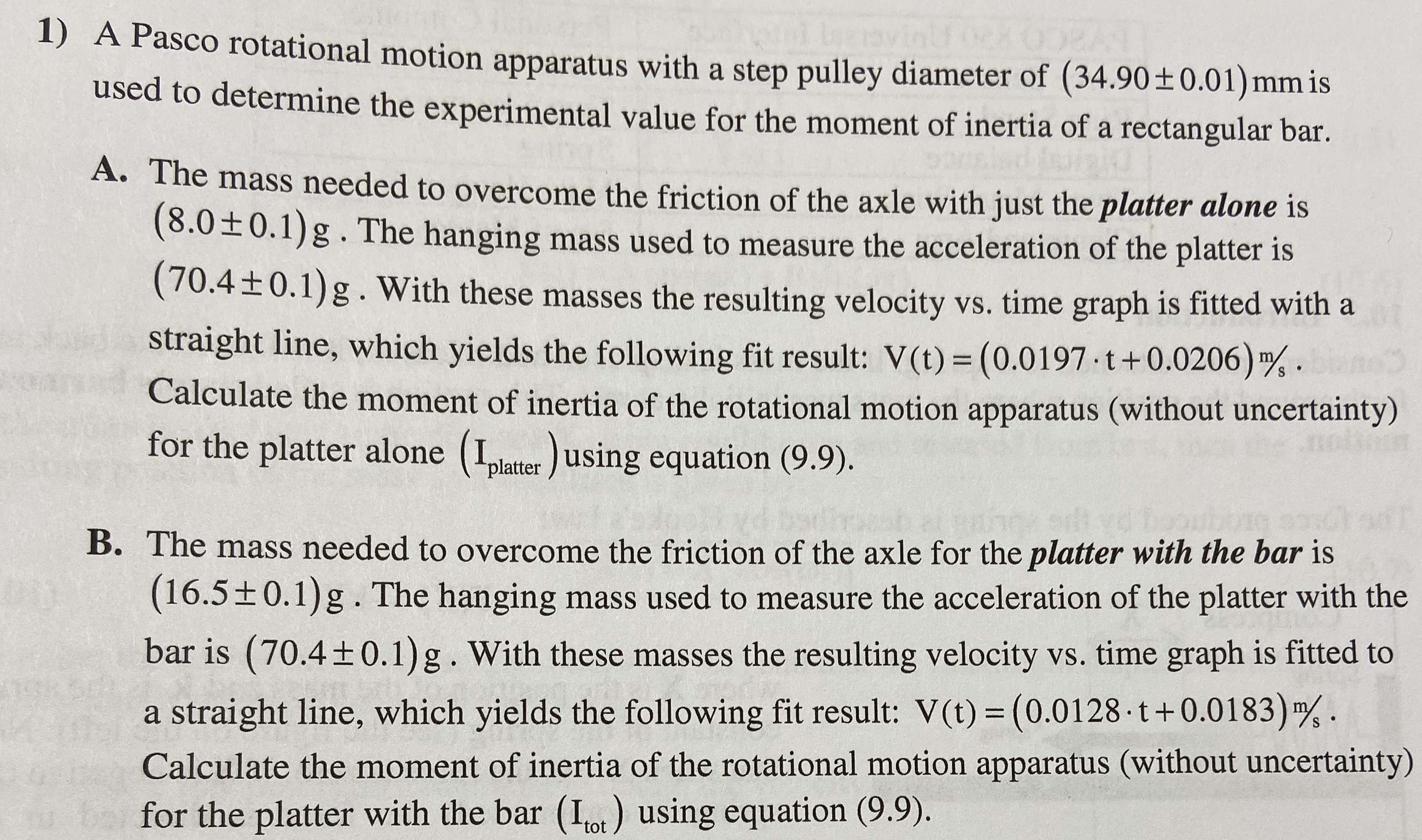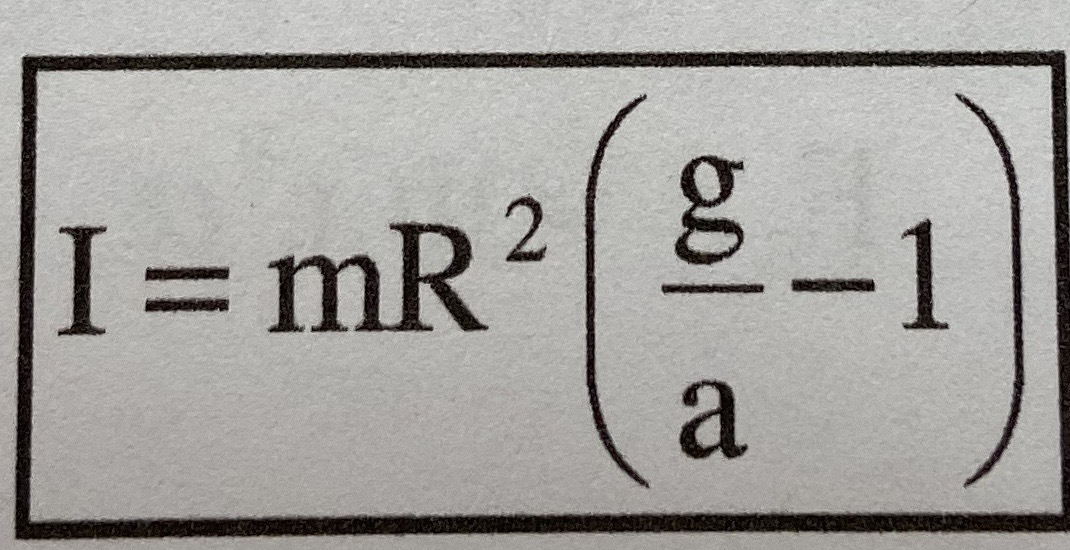# 1) A Pasco rotational motion apparatus with a step pulley diameter of (34.90 ± 0.01) mm isused to determine the experimental value for the moment of inertia of a rectangular barA. The mass needed to overcome the friction of the axle with just the platter alone is(8.0±0.1)g.The hanging mass used to measure the acceleration of the platter is(70.4+0.1) g. With these masses the resulting velocity vs. time graph is fitted with astraight line, which yields the following fit result: V(t) (0.0197 t+0.0206) 4Calculate the moment of inertia of the rotational motion apparatus (without uncertainty)for the platter alone (plater using equation (9.9).Se sB. The mass needed to overcome the friction of the axle for the platter with the bar is(16.5±0.1)g.The hanging mass used to measure the acceleration of the platter with thebar is (70.40.1)g . With these masses the resulting velocity vs. time graph is fitted tostraight line, which yields the following fit result: V(t) (0.0128 t + 0.01 83)Calculate the moment of inertia of the rotational motion apparatus (without uncertainty)bafor the platter with the bar (Iot) using equation (9.9) I= mR2-1a

Question
18 viewshelp_outlineImage Transcriptionclose1) A Pasco rotational motion apparatus with a step pulley diameter of (34.90 ± 0.01) mm is used to determine the experimental value for the moment of inertia of a rectangular bar A. The mass needed to overcome the friction of the axle with just the platter alone is (8.0±0.1)g.The hanging mass used to measure the acceleration of the platter is (70.4+0.1) g. With these masses the resulting velocity vs. time graph is fitted with a straight line, which yields the following fit result: V(t) (0.0197 t+0.0206) 4 Calculate the moment of inertia of the rotational motion apparatus (without uncertainty) for the platter alone (plater using equation (9.9). S e s B. The mass needed to overcome the friction of the axle for the platter with the bar is (16.5±0.1)g.The hanging mass used to measure the acceleration of the platter with the bar is (70.4 0.1)g . With these masses the resulting velocity vs. time graph is fitted to straight line, which yields the following fit result: V(t) (0.0128 t + 0.01 83) Calculate the moment of inertia of the rotational motion apparatus (without uncertainty) bafor the platter with the bar (Iot) using equation (9.9) fullscreenhelp_outlineImage TranscriptioncloseI= mR2 -1 a fullscreen
check_circle

Step 1

The total moment of...

### Want to see the full answer?

See Solution

#### Want to see this answer and more?

Solutions are written by subject experts who are available 24/7. Questions are typically answered within 1 hour.*

See Solution
*Response times may vary by subject and question.
Tagged in

### Science